• 模糊聚类分析实验报告+matlab代码 模糊聚类分析实验报告+matlab代码
• 通过matlab对fcm模糊聚类分析进行了代码实现。代码简单适合初学者。
• 模糊聚类分析matlab程序实现 采用模糊数学语言对按一定的要求进行描述和分类的数学方法称为模糊聚类分析聚类分析主要经过标定和聚类两步骤1 1 标定建立模糊相似矩阵 城市居民食品零售价格第t时刻第i种食品的零售价...
• 运用matlab进行模糊聚类分析步骤如下：建立数据矩阵；数据标准化；建立模糊相似矩阵；改造相似关系为等价关系；确定分类数
• 可疑分析matlab源代码GBK均值聚类算法 欢迎。 该存储库包含基于 matlab 的“GBK-means 聚类算法：基于讨价还价游戏的 K-means 算法的改进”的实现。 在这个存储库中，介绍了 GBK-means 聚类算法的源代码及其与两个...
• 1 基于Matlab模糊聚类分析及其应用 管理数学实验课程汇报 学号2120111705 姓名贾珊 预备知识 1 基于MATLAB模糊聚类分析的传递方法 2 实例应用 3 Contents 3 1.预备知识 1.预备知识 聚类分析和模糊聚类分析 模糊...
• %模糊聚类程序 function f=mujl(x,lamda) %输入原始数据以及lamda的值 if lamda>1 disp('error!') %错误处理 end [n,m]=size(x); xmax=max(x);xmin=min(x); x=(x-xmin(ones(n,1),:))./(xmax(ones(n,1),:)-xmin...
• ## python/matlab进行模糊聚类分析

千次阅读 多人点赞 2019-09-30 18:12:11
思路 根据下面表格中的数据，用Matlab（或Python）...根据模糊等价矩阵，编程绘制动态聚类图； 根据原始数据，编程确定最佳分类结果。 原始数据如下： no Y1 Y2 Y3 Y4 Y5 Y6 x1 21 63 19 40 1.567 106 x2 23 74 ...
问题
根据下面表格中的数据，用Matlab（或Python）编程进行数据标准化处理；根据标准化处理后的数据，用Matlab（或Python）编程，建立模糊相似矩阵，并编程求出其传递闭包矩阵；根据模糊等价矩阵，编程绘制动态聚类图；根据原始数据，编程确定最佳分类结果。 原始数据如下： no Y1 Y2 Y3 Y4 Y5 Y6 x1 21 63 19 40 1.567 106 x2 23 74 30 75 2.693 54 x3 119 179 86 118 6.897 9 x4 115 168 49 89 2.637 29 x5 79 146 46 92 2.356 24 x6 79 158 48 103 2.142 7 x7 65 114 58 99 2.679 7 x8 68 119 58 96 3.099 6 x9 109 166 59 95 2.868 6 x10 118 177 56 89 2.64 7
代码如下
# -*- coding: utf-8 -*-
import numpy as np
import pprint
import xlrd
from copy import deepcopy
import math
from scipy.cluster import hierarchy  #用于进行层次聚类，话层次聚类图的工具包
from scipy import cluster
import matplotlib.pyplot as plt
import pandas as pd
#矩阵不用科学计数法的显示方式
np.set_printoptions(suppress=True)

# 1.数据规范化：采用最大值规格法
def process_matrix(ori_matrix):
x = deepcopy(ori_matrix)
#获得特征指标矩阵行列数
x_rows = x.shape#10,行数
x_cols = x.shape#6，列数
#参数0代表对每一列求均值，参数1代表对每一行求均值，无参数则求所有元素的均值
max_matrix = np.max(x,axis = 0)
for i in range(x_rows):
for j in range(x_cols):
x[i][j] = (x[i][j])/max_matrix[j]
return x

# 2.构造模糊相似矩阵：采用最大最小法构造模糊相似矩阵
def get_rmatrix(Ori_matrix):

# Fuzzy_Similarity_Matrix : 模糊相似矩阵
Fuzzy_Similarity_Matrix = np.zeros((Ori_matrix.shape,Ori_matrix.shape),dtype = 'float')

for i in range(Ori_matrix.shape):
for j in range(Ori_matrix.shape):
max_sum = 0
min_sum = 0
for k in range(Ori_matrix.shape):
max_sum += max(Ori_matrix[i][k], Ori_matrix[j][k])
min_sum += min(Ori_matrix[i][k], Ori_matrix[j][k])
Fuzzy_Similarity_Matrix[i][j] = min_sum/max_sum

return Fuzzy_Similarity_Matrix

# 3.求传递闭包
def get_tR(r_matrix):
def t_r(mat):#传递闭包运算
rows = mat.shape
cols = mat.shape
min_list = []
new_mat = np.zeros((rows,cols),dtype = 'float')
for m in range(rows):
for n in range(cols):
min_list = []
now_row = mat[m]
now_col = mat[:,n]
for k in range(len(now_row)):
min_cell = min(mat[m][k],mat[:,n][k])
min_list.append(min_cell)
new_mat[m][n] = max(min_list)
return new_mat
#传递闭包判断是否停止运算
i = 0
while True:
t_r_matrix = t_r(r_matrix)
print("==================模糊相似矩阵R^%d================"%(2**i))
print(t_r_matrix)
#若get_tR(r_matrix) == t_r_matrix ，退出循环
if (t_r_matrix == r_matrix).all():
print("********  R^%d = R^%d ,传递闭包过程结束。******** "%(2**(i-1),2**i))
break
#否则，将t_r_matrix作为待求闭包矩阵
else:
r_matrix = t_r_matrix
i += 1
return t_r_matrix

# 4.按lambda截集进行动态聚类
def lambda_clustering(final_matrix):
rows = final_matrix.shape
cols = final_matrix.shape
lambda_list = list(set([i for j in final_matrix.tolist() for i in j]))#将numpy数组转化为一维列表并去重得到截集
lambda_list.sort(reverse=True)
result = [] #返回的结果
for i in range(len(lambda_list)):
class_list = []  #分类情况
temp_infor = {'matrix':np.zeros((rows,cols),dtype = 'float'),'lambda':lambda_list[i],'class':[]} #每个lambda值的分类情况

for m in range(rows):#计算大于等于截集值的置1，小于截集值置0
for n in range(cols):
if final_matrix[m][n] >= lambda_list[i]:
temp_infor['matrix'][m][n] = 1
else:
temp_infor['matrix'][m][n] = 0

for m in range(rows):#首先遍历每一行，设这一行为m，与下一行即下一个实例比较
if (m+1) in [i for item in class_list for i in item]:#如果 m+1 行的索引号在 已经分好类的所有实例(class_list) 中就跳出循环，即 m+1 行的那个实例已经被分类完了
continue
now_class = []#如果没跳出循环说明这个m+1这个实例还没被分类，now_class用来存储 第m+1 个实例和其后面相同类别的实例
now_class.append(m+1)
for n in range(m+1,rows):#对于m+1及其往后的实例，若有相等归为一类向now_class添加元素
if (temp_infor['matrix'][m] == temp_infor['matrix'][n]).all() :
now_class.append(n+1)
class_list.append(now_class)

temp_infor['class'] = class_list
result.append(temp_infor)
return result

# 5.利用 F - 统计量寻找最优分类
#传入参数为result动态聚类结果字段有class（分类情况），lamdba（截集取值），matrix（0-1矩阵）standard_matrix标准化后的矩阵
def F_Statistics(results, standard_matrix):
#定义变量F 为F统计量数值    #定义class_class变量为类与类之间的距离  F的分子#    定义class_sample变量为类内样品间的距离  F的分母
for result in results:
mean_all = np.mean(standard_matrix, axis=0)#计算矩阵所有样品属性的平均值
sorts = result.get('class')
class_class = 0
class_sample = 0
for sort in sorts:#对于每个分类
sort = [i-1 for i in sort]#将分类转化 [4, 9, 10]-->[3, 8, 9]因为矩阵下标从零开始
# print(standard_matrix[sort])
mean_j = np.mean(standard_matrix[sort], axis=0)#计算矩阵第j类样品的平均值
xj_av_reduce_xall_av = (np.sum((np.square(mean_j - mean_all)), axis=0))**(1/2)#计算xj平均值减去x的平均值,对所有属性
class_class += (len(sort) * xj_av_reduce_xall_av**2)

#[nan, 138.72123087068982, 91.13931639141123, 33.60939825599838, 37.53752831809482, 18.76906273985417, 12.97829257460103, 7.850374240762219, 13.852860873165161, inf]
#计算类class_sample 为类内样品间的距离
sum_xij_reduce_xj_av = 0#用来储存这个类中所有样品的xij_reduce_xj_av值
for s in sort:#求每一个样品的xij_reduce_xj_av，对所有属性
xij_reduce_xj_av = (np.sum(np.square((standard_matrix[s] - mean_j)), axis=0))**(1/2)#计算x(i,j)减去xj的平均值，对所有属性
sum_xij_reduce_xj_av += (xij_reduce_xj_av**2)
class_sample += sum_xij_reduce_xj_av
F = (class_class / (len(sorts) - 1)) / (class_sample / (standard_matrix.shape - len(sorts)))
result['F'] = F

print("********************   对于上面抛出警告 RuntimeWarning: divide by zero encountered in double_scalars 忽略    ************************")
F_np = np.array([result.get('F') for result in results])
where_are_inf = np.isinf(F_np)#无穷大的值组成的布尔列表
where_not_inf = (1-where_are_inf).astype(np.bool)#对上面取反，得到不是无穷大的正常值
F_np[where_are_inf] = np.mean(F_np[where_not_inf])#将无穷大值的地方置为平均值

where_are_nan = np.isnan(F_np)#nan的值组成的布尔列表
where_not_nan = (1-where_are_nan).astype(np.bool)#对上面取反，得到不是nan的正常值
F_np[where_are_nan] = np.mean(F_np[where_not_nan])#将nan值的地方置为平均值,原谅我不会布尔矩阵取或运算
print("***********最优分类结果如下***************")
return np.argmax(F_np)#返回F取最大时候的下标

def main():
# 0.读取数据
path = 'data.xlsx'
ori_matrix = df.values

# 1.数据规范化：采用最大值规格法规范化原矩阵
standard_matrix = process_matrix(ori_matrix)

# 2.构造模糊相似矩阵：采用最大最小法构造模糊相似矩阵
r_matrix = get_rmatrix(standard_matrix)

#  将模糊相似矩阵取两位小数，否则后面的与lambda值判断大小时会出错
for i in range(r_matrix.shape) :
for j in range(r_matrix.shape) :
r_matrix[i][j] = round(r_matrix[i][j],2)

print('==================特征指标矩阵================')
print(ori_matrix)
print('===============标准化特征指标矩阵=============')
print(standard_matrix)
print('==================模糊相似矩阵R================')
print(r_matrix)

# 3.求传递闭包t(R)
t_r_matrix = get_tR(r_matrix)

Z = hierarchy.linkage(t_r_matrix, method ='ward', metric='euclidean')
hierarchy.dendrogram(Z,labels = df.index)
label = cluster.hierarchy.cut_tree(Z,height=1)
label = label.reshape(label.size,)
plt.show()

# 4.按lambda截集进行动态聚类
result = lambda_clustering(t_r_matrix)
print("************动态聚类结果为****************")
for x in result:
pprint.pprint(x)
# 5.利用 F - 统计量寻找最优分类
pprint.pprint(result[F_Statistics(result,standard_matrix)])
if __name__ == '__main__':
main()


参考
https://blog.csdn.net/CodertypeA/article/details/86483175 https://blog.csdn.net/m0_37804518/article/details/78806138
-----------------------------------------------------------matlab----------------------------------------------------------------------
matlab版模糊聚类分析
Main.m
x=[37 38 12 16 13 12;
69 73 74 22 64 17;
73 86 49 27 68 39;
57 58 64 84 63 28;
38 56 65 85 62 27;
65 55 64 15 26 48;
65 56 15 42 65 35 ;
66 45 65 55 34 32];
x_zuida = [];
x_pingyi = [];
R_zuidazuixiao = [];
R_suanshu = [];
[x_zuida,x2] = bzh(x);
[R_zuidazuixiao,R_suanshu] = bd(x_zuida);
tR_zuidazuixiao = chuandi(R_zuidazuixiao)
tR_suanshu = chuandi(R_suanshu)
juleitu(tR_zuidazuixiao)
julei(tR_zuidazuixiao)


bd.m
function [R1,R2] = bd(x)
%函数功能：标定
[m,n] = size(x);
for i = 1:m
for j = 1:m
for k = 1:n
qx(k) = min(x(i,k),x(j,k));  %取小
qd(k) = max(x(i,k),x(j,k));   %取大

end
R1(i,j) = sum(qx)/sum(qd);  %最大最小法
R2(i,j) = 2*sum(qx)/(sum(x(i,:))+sum(x(j,:)));  %算术平均最小法
if i == j
R1(i,j) = 1;
R2(i,j) = 1;
end
end
end
R_zuidazuixiao = R1
R_suanshu = R2


bzh.m
function [x_zuida, x_pingyi] = bzh(x)
%函数功能：标准化矩阵
[m,n] = size(x);
B = max(x);
B1 = max(x) - min(x);
Bm = min(x);
for i = 1:n
x1(:,i) = x(:,i)/B(i);  %最大值规格化
x2(:,i) = (x(:,i) - Bm(i))/B1(i);  %平移极差标准化
end
x_zuida = x1
x_pingyi = x2


chuandi.m
function [tr] = chuandi(x)
%函数功能：求传递闭包
R = x;
a=size(R);
B=zeros(a);
flag=0;
while flag==0
for i= 1: a
for j= 1: a
for k=1:a
B( i , j ) = max(min( R( i , k) , R( k, j) ) , B( i , j ) ) ;%R与R内积，先取小再取大
end
end
end
if B==R
flag=1;
else
R=B;%循环计算R传递闭包
end
end
tr = B;


julei.m
function [M,N]=julei(tR1)
%函数功能：求出lamda截矩阵
tR = tR1;
lamda=unique(tR);  %取A矩阵不同元素构成的向量，来确定阈值
L=length(lamda);
lamda = sort(lamda,'descend');
for i = 1:L
tR = tR1;
lamda(i)
tR(find(tR>=lamda(i))) = 1;  %令大于lamda的为1
tR(find(tR<lamda(i))) = 0;  %令小于lamda的为0
tR
end


juleitu.m
function [M,N]=juleitu(tR)
%函数功能：画动态聚类图
lamda=unique(tR);%取A矩阵不同元素构成的向量，来确定阈值
L=length(lamda);
M=1:L;
for i=L-1:-1:1  %获得分类情况：对元素分类进行排序
[m,n]=find(tR==lamda(i));
N{i,1}=n;
N{i,2}=m;
tR(m(1),:)=0;
mm=unique(m);
N{i,3}=mm;
len=length(find(m==mm(1)));
depth=length(find(m==mm(2)));
index1=find(M==mm(1));
MM=[M(1:index1-1),M(index1+depth:L)];
index2=find(MM==mm(2));
M=M(index1:index1+depth-1);
M=[MM(1:index2-1),M,MM(index2:end)];
end
M=[1:L;M;ones(1,L)];
h=(max(lamda)-min(lamda))/L;
figure
text(L,1,sprintf('x%d',M(2,L)));
text(0,1,sprintf('%3.4f',1));
text(0,(1+min(lamda))/2,sprintf('%3.4f',(1+min(lamda))/2));
text(0,min(lamda),sprintf('%3.4f',min(lamda)));
hold on
for i=L-1:-1:1    %获得分类情况：每一个子类的元素
m=N{i,2};
n=N{i,1};
mm=N{i,3};
k=find(M(2,:)==mm(1));
l=find(M(2,:)==mm(2));
x1=M(1,k);
y1=M(3,k);
x2=M(1,l);
y2=M(3,l);
x=[x1,x1,x2,x2];
M(3,[k,l])=lamda(i);
M(1,[k,l])=sum(M(1,[k,l]))/length(M(1,[k,l]));
y=[y1,lamda(i),lamda(i),y2];
plot(x,y);
text(i,1,sprintf('x%d',M(2,i)));
text(M(1,k(1)),lamda(i)+h*0.1,sprintf('%3.4f',lamda(i)));
end
axis([0 L+1 min(lamda) max(lamda)])
axis off
hold off
end


将main函数里的矩阵改一下就能用
展开全文• 运用MATLAB模糊聚类系谱图，该方法简便，动态谱系图具有多个分类系数与多种分析，可以按需选择
• Matlab实现模糊聚类分析 FCM 模糊均值聚类（FCM）是用隶属度确定每个数据点属于某个聚类的程度的一种聚类算法。1973年，Bezdek提出了该算法，作为早期硬均值聚类（HCM）方法的一种改进。FCM把 n 个向量 xi(i=1,2,…...
Matlab实现模糊聚类分析 FCM
模糊均值聚类（FCM）是用隶属度确定每个数据点属于某个聚类的程度的一种聚类算法。1973年，Bezdek提出了该算法，作为早期硬均值聚类（HCM）方法的一种改进。FCM把 n 个向量 xi(i=1,2,…,n)分为 c 个模糊组,并求每组的聚类中心，使得非相似性指标的价值函数达到最小。FCM 使得每个给定数据点用值在 0,1 间的隶属度来确定其属于各个组的程度。与引入模糊划分相适应,隶属矩阵 U 允许有取值在 0,1 间的元素。不过,加上归一化规定，一个数据集的隶属度的和总等于 1: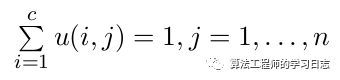那么，FCM的价值函数（或目标函数）：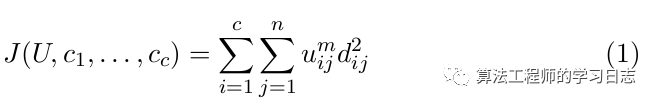这里 uij 介于 0，1 间；ci 为模糊组 i 的聚类中心，dij=||ci-xj||为第 i 个聚类中心与第 j 个数据点间的欧几里德距离；且 m （属于1到无穷） 是一个加权指数。 构造如下新的目标函数，可求得使下式达到最小值的必要条件:其实就是拉格朗日乘子法：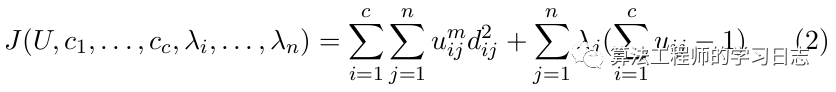对上式所有输入参量求导，使上式达到最小的必要条件为: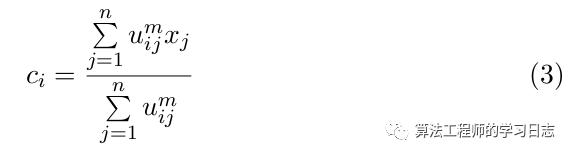和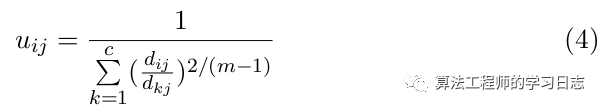由上述两个必要条件，模糊 C 均值聚类算法是一个简单的迭代过程。在批处理方式运行时, FCM 用下列步骤确定聚类中心 ci 和隶属矩阵 U: 步骤 1:用值在 0,1 间的随机数初始化隶属矩阵 U 步骤 2:用式(3)计算 c 个聚类中心 ci,i=1,…,c。 步骤 3:根据式(1)计算价值函数。如果它小于某个确定的阀值，或它相对上次价 值函数值的改变量小于某个阀值，则算法停止。 步骤 4:用(4)计算新的 U 矩阵和。返回步骤 2。 上述算法也可以先初始化聚类中心，然后再执行迭代过程。由于不能确保 FCM 收敛于一个最优解。算法的性能依赖于初始聚类中心。因此,我们要么用另外的快速算法确定初始聚类中心，要么每次用不同的初始聚类中心启动该算法，多次运行 FCM。
注：上面讨论不难看出二个参数比较重要：1.聚类的数目，2.控制算法的参数m，如果m过大，则聚类的效果很差，如果m过小，则算法接近Kmeans算法。
借用Matlab自带的fcm函数来做个例子
data = rand(100,2); % 数据
options = [2;100;1e-5;1]; % 控制参数
[center,U,obj_fcn] = fcm(data,2,options);  % 聚类为2类
figure;
plot(data(:,1), data(:,2),'o');
title('Demo');
grid on;
hold on;
maxU = max(U);
index1 = find(U(1,:) == maxU);
index2 = find(U(2,:) == maxU);
line(data(index1,1),data(index1,2),'marker','*','color','g');
line(data(index2,1),data(index2,2),'marker','*','color','r');
plot([center([1 2],1)],[center([1 2],2)],'*','color','k')
hold off;
figure
plot(obj_fcn)
title('Objective Function Values')
xlabel('Iteration Count')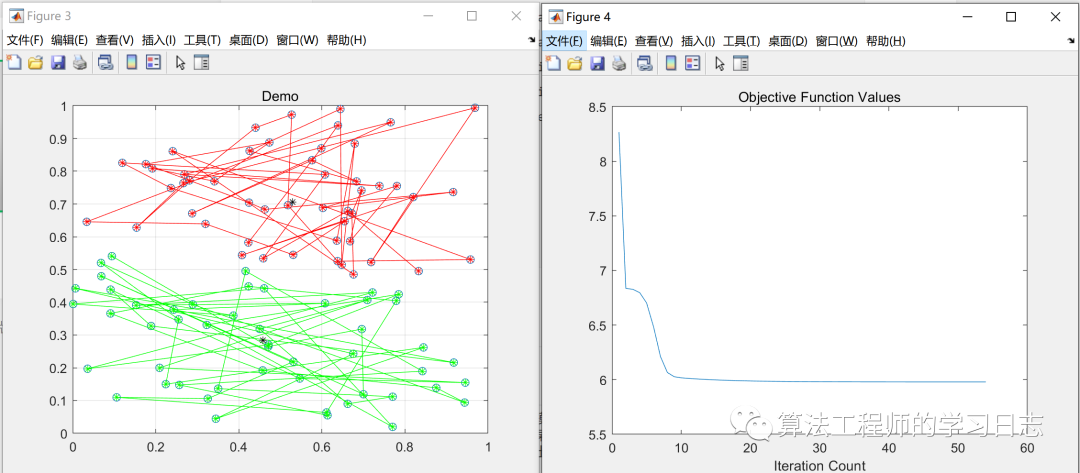展开全文接上上篇文章：数据分析模型（二）：模糊聚类分析方法及实例（附完整代码）
主要对代码里面的用到的主要函数进行详细讲解，同时也会对利用MATLAB对数据分析有质的飞跃！！！欢迎关注学习！！！

Matlab中关于模糊聚类分析的函数

1）pdist 计算两两对象间的欧氏距离
Y=pdist(X)计算 m × n 矩阵X（看作 m 个 n 维行向量）中两两对象间的欧氏距离。对于有 m 个对象组成的数据集，共有(m-1)⋅ m/2个两两对象组合。
输出Y是包含距离信息的长度为 (m-1)⋅ m/2的向量。可用squareform函数将此向量转换为方阵，这样可使矩阵中的元素（i,j）对应原始数据集中对象i和j间的距离。
Y=pdist(X,’metric’)中用’metric’指定的方法计算矩阵X中对象间的距离。’metric’可取表1中特征字符串值。
表 1’metric’取值及含义
字符串含 义’Euclid’欧氏距离（缺省）’SEuclid’标准欧氏距离’Mahal’马氏距离（Mahalanobis距离）’CityBlock’绝对值距离’Minkowski’闵氏距离（Minkowski距离）
Y=pdist(X,’minkowski’,p)用闵氏距离计算矩阵X中对象间的距离。p为闵氏距离计算用到的指数值，缺省为2。
Z=linkage(Y)使用最短距离算法生成具层次结构的聚类树。输入矩阵Y为pdist函数输出的 (m-1)⋅ m/2维距离行向量。
Z=linkage(Y, 'method')使用由’method’指定的算法计算生成聚类树。'method’可取表2中特征字符串值。
表2 ’method’取值及含义
字符串含 义’single’最短距离（缺省）’complete’最大距离’average’平均距离’centroid’重心距离’ward’离差平方和方法（Ward方法）
输出Z为包含聚类树信息的(m-1) × 3 矩阵。聚类树上的叶节点为原始数据集中的对象，由1到 m 。它们是单元素的类，级别更高的类都由它们生成。对应于Z中行 j 每个新生成的类，其索引为 m+j ，其中 m 为初始叶节点的数量。
第1列和第2列，即Z(i,1:2)包含了被两两连接生成一个新类的所有对象的索引。生成的新类索引为 m+j 。共有 m-1 个级别更高的类，它们对应于聚类树中的内部节点。第3列，Z(i,3)包含了相应的在类中的两两对象间的连接距离。
T=cluster(Z,cutoff)从连接输出（linkage）中创建聚类。cutoff为定义cluster 函数如何生成聚类的阈值，其不同的值含义如表3所示。
表3 cutoff取值及含义
cutoff取值含 义0<cutoff<2cutoff作为不一致系数的阈值。不一致系数对聚类树中对象间的差异进行了量化。如果一个连接的不一致系数大于阈值，则cluster函数将其作为聚类分组的边界2<=cutoffcutoff作为包含在聚类树中的最大分类数
T=cluster(Z,cutoff,depth,flag)从连接输出(linkage)中创建聚类。参数depth 指定了聚类数中的层数，进行不一致系数计算时要用到。不一致系数将聚类树中两对象的连接与相邻的连接进行比较。详细说明见函数inconsistent。当参数depth被指定时，cutoff通常作为不一致系数阈值。
参数flag重载参数cutoff的缺省含义。如flag为’inconsistent’，则cutoff作为 不一致系数的阈值。如flag为’cluster’，则cutoff作为分类的最大数目。
输出T为大小为 m 的向量，它用数字对每个对象所属的类进行标识。为了找到包含在类i中的来自原始数据集的对象，可用find(T==i)。
4）zsore(X) 对数据矩阵进行标准化处理
对数据矩阵进行标准化处理，处理方式为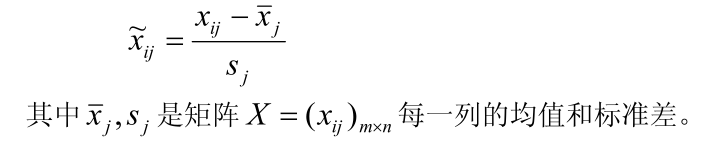5）H＝dendrogram(Z,P) 画聚类树状图
6）T=clusterdata(X,cutoff) 将矩阵X的数据分类
将矩阵X的数据分类。X为 m×n 矩阵，被看作 m 个 n 维行向量。它与以下几个命令 等价：
Y=pdist(X,’euclid’)
T=cluster(Z,cutoff)

7) squareform 将pdist的输出转换为方阵
将pdist的输出转换为方阵。
8）cophenet 计算相干系数
c=cophenet(Z,Y) 计算相干系数，它是将Z中的距离信息（由linkage()函数产生）和Y中的距离信息（由pdist()函数产生）进行比较。Z为 (m-1) × 3 矩阵，距离信息包含在第三列。Y是(m-1) ⋅m/2 维的行向量。
展开全文• 模糊聚类的一个小例子，自编模糊聚类代码，供参考使用。
• ## 聚类分析Matlab代码

千次阅读 2019-08-19 20:55:49
%聚类分析代码 clc,clear load demo2.txt %把原始数据保存在纯文本文件demo2.txt中 demo2(:,[3:6])=[]; %删除数据矩阵的第3列～第6列,即使用变量1,2,7,8,9,10 demo2=zscore(demo2); %数据标准化 y=pdist...
%聚类分析代码
clc,clear
demo2(:,[3:6])=[]; 					%删除数据矩阵的第3列～第6列,即使用变量1,2,7,8,9,10
demo2=zscore(demo2); 		%数据标准化
y=pdist(demo2); 					%求对象间的欧氏距离,每行是一个对象
h=dendrogram(z); 				 %画聚类图
xlabel('城市编号');
ylabel('分类结果');
set(h,'Color','b','LineWidth',1.3)  			%把聚类图线的颜色改成蓝色，线宽加粗
for k=3:5
fprintf('划分成%d类的结果如下：\n',k)
T=cluster(z,'maxclust',k);  				%把样本点划分成k类
for i=1:k
tm=find(T == i);  								%求第i类的对象
tm=reshape(tm,1,length(tm)); 		%变成行向量
fprintf('第%d类的有%s\n',i,int2str(tm)); 				%显示分类结果
end
if k==5
break
end
fprintf('**********************************\n');
end

展开全文数学建模
• 为了对不同水害类型的矿井进行分类以制定相应的防治水措施,选取矿井涌水量、突水量以及主要含水层单位涌水量作为聚类指标,基于MATLAB模糊聚类分析法将研究的11个矿井进行分类。结合水文地质条件可将矿井分为4类,第...
• 基于MATLAB模糊聚类分析的中传递方法的描述
• 模糊聚类分析是涉及事物之间的模糊界限时按一定要求对事物进行分类的数学方法。聚类分析是数理统计中的一种多元分析方法，它是用数学方法定量地确定样本的亲疏关系，从而客观地划分类型。事物之间的界限，有些是确..
• 模糊聚类分析matlab 程序实现 采用模糊数学语言对按一定的要求进行描述和分类的数学方法称为模糊聚 1 类分析聚类分析主要经过标定和聚类两步骤 1 标定建立模糊相似矩阵 城市居民食品零售价格第 t 时刻第 i 种食品...
• 对于Iris数据集，使用模糊聚类及模糊推理系统对其进行分类模糊系统 机器学习 鸢尾花Iris
• 简单介绍模糊聚类原理，并对算法进行实际案例讲解。
• 模糊聚类小例子,模糊聚类分析例题,matlab源码
• 基于MATALAB的数据挖掘聚类分析算法之一：模糊聚类分析算法示例模板，希望对大家有帮助
• ## 模糊聚类分析

千次阅读 2018-12-16 22:34:15
文章目录基于模糊等价矩阵的聚类分析方法建立模糊矩阵建立模糊等价矩阵建立模糊相似矩阵数据预处理——数据标准化平移－极差变换（变换至0-1区间）平移－标准差变换（消除量纲）模糊相似矩阵的建立相似系数法数量积...
• ## 模糊聚类FCM的MATLAB实现

万次阅读 多人点赞 2016-03-29 21:56:46
• 模糊聚类分析原理及实现 聚类分析就是用数学方法研究和处理所给定对象 按照事物间的相似性进行区分和分类的过程 传统的聚类分析是一种硬划分 它把每个待识别的对象严格地划分到某个类中具有非此即彼的性质 这种分类...
• 利用模糊聚类分析编程 matlab作为编程的工具，具有界面友好使用方便，运行良好的，快速的优点 ，可以方便的进行各种各样的数据的模糊聚类分析
• 模糊c-均值(FCM)聚类算法在matlab中实现,已测试通过
• ## 聚类分析matlab

万次阅读 多人点赞 2016-09-05 15:09:14
聚类与分类的不同就是，聚类所要求的划分的类是未知的。  聚类是这么定义的：将数据分类到不同的类或者簇这样的一个过程，所以同一个簇中的对象有很大的相似性，而不同簇之间的对象很大的相异性...

# 模糊聚类分析matlabmatlab 订阅